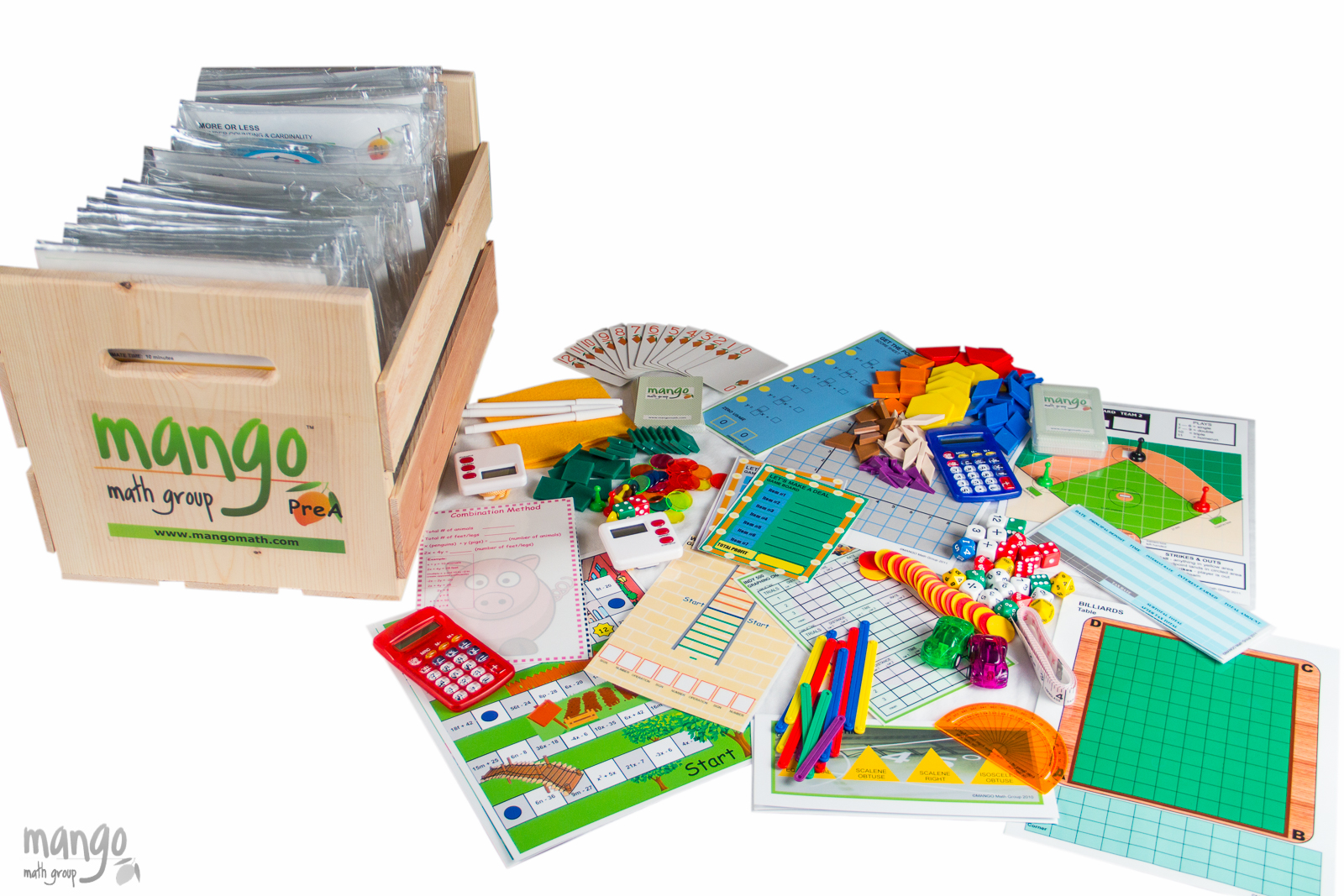#### Order Notice

As the economy opens back up, many of our suppliers are experiencing shortages of parts and raw materials, resulting in delays in shipments. As a result, we anticipate that it may take up to 2-4 weeks from the date we receive a purchase order for us to fulfill and ship any order for our products. We apologize for any inconvenience.

# Pre-Algebra Math Kit

Pre-Algebra made fun and easy! MANGO Math's Strong Evidence Based Pre-Algebra Math kit is designed specifically for grades 6-7-8. The kit contains 25 separate skill building math lessons.

\$ 459.00 USD##### Product Details

Pre-Algebra made fun and easy! MANGO Math Pre-Algebra Math kit is designed specifically for grades 6-7-8. The kit contains 25 separate skill building math lessons.

Each individual lesson offers visual and kinetic representation of math concepts that develop strong math knowledge. like:

• ratio
• fractions
• percent
• integers
• expressions
• factors
• exponents

Every lesson is based on a game or activity that fosters curiosity, discussion and growth through cooperation, collaboration and creative thinking.

MANGO Math's Strong Evidence Based Kits come with individually packaged, Grab-n-Go lessons that includes instructions, reusable materials pre-packaged in a resealable pouch contained in an easy to carry crate that can be used year after year, providing long term savings.

A lesson can be used by up to 4 students, set up as math centers with each grade level math kit meeting the needs of 30+ students at a time.

Each MANGO Math grade level math kit is research-based and adheres to Common Core, TEKS and NCTM math standards.

Great to use in regular classrooms, resource rooms, after school programs, STEM programs, libraries, math centers, summer camps, learning cooperatives, math clubs, community centers, and at home.

#### All the supplies below are included with its specific lesson in the crate:

• Spinners
• 6-sided dice
• 10-sided dice
• 12-sided dice
• 20-sided dice
• 7 – 12 number dice
• Positive/negative dice
• Tens dice
• Thousands dice
• Bi-colored counters
• Transparent counters
• Colored tiles
• Anglegs
• Measuring tape
• Timers
• Calculators
• MANGO Math playing cards
• Friction cars
• Fraction pattern blocks
• Game pawns
• Plastic resealable pouches
• Game boards
• Laminated writing boards
• Dry erase markers
• Easy to Follow Direction cards
• Curriculum Guide

## Pre-Algebra (Grades 6, 7, 8) Lessons with Standards

Billiards – Measurement & Proportional Relationships: Recognize and represent proportional relationships between quantities.  Decide whether two quantities are in a proportional relationship.

Dots & Boxes – Ratios: Understand the concept of a ratio and use ratio language to describe a ratio relationship.

Mini Me – Ratios & Proportional Relationships: Recognize and represent proportional relationship between quantities.

Tisk Task – Ratios: Compute unit rates associated with ratios of fractions.

Factorland – Expressions: Apply properties of operations as strategies to add, subtract factors and expand linear expressions with rational coefficients.

Get My Point – Linear Equations: Understand the connections between proportional relationships, lines and linear equations.

Great Expressions – Equations: Write, read and evaluate expression in which letters stand for numbers.

Bracket Play – Expressions & Equations: Apply the properties of operations to generate equivalent expressions.

Indy 500 – Proportional Relationships: Use variables to represent two quantities in a real-world problem that change in relationship to one another. Compare two proportional relationships.

Interest-ed – Expressions & Equations: Use variable to represent numbers and write expressions when solving  multiple step real-world problems.

Let’s Make a Deal – Equations: Find a percent of a quantity as a rate per 100, solve problems involving finding the whole, given a part and the percent.

Math Magic – Expression & Equations: Use variables to represent quantities and construct simple equations and inequalities to solve problems by reasoning.

Pigs and Penguins – Expression & Equations: Analyze and solve pairs of simultaneous linear equations.

Prime Time – Expression & Equations: Use square root and cube root symbols to represent solutions to equations of the form x2 = P and x3 = p.

All Things Equal – Number Sense: Understand the multiplication and division particularly the distributive property.

Bottoms Up – Number Sense: Interpret and compute quotients of fractions involving division of fraction by fractions.

Nim – Mathematical Practice: Reason abstractly, make sense of problems and persevere, Look for and express regularity in repeated reasoning.

Taxicab Treasure – Number Sense: Understand signs of numbers in ordered pairs as indicating location in quadrants of the coordinate plane.

What’s Your Sign – Number Sense: Apply and extend understanding of addition and subtraction, multiplication and division  to understanding integers.

Zilch – Number Sense: Understand that positive and negative numbers are used together to describe quantities having opposite direction or value and comparison of absolute value.

Pythagorean Baseball – Geometry: Apply the Pythagorean Theorem to determine unknown side lengths in right triangles.

Taking Up Space – Geometry:  Solve real-life and mathematical problems involving angle measure, area, surface area, and volume.

Triangle Techie – Geometry: Draw geometric shapes with given conditions.  Focus on constructing triangles from three measurement of angles or sides.

Scatter Plot Boot Camp – Statistics & Probability: Construct and interpret scatter plots for bivariate measurement data.

Sticks & Stones – Statistics & Probability: Approximate the probability of a chance event by collecting data on the chance process that produces it.

Width
13
in
Height
13
in
Length
19
in
Weight
20
lb
{% if context == "cart" or context == "checkout" %} {% endif %}